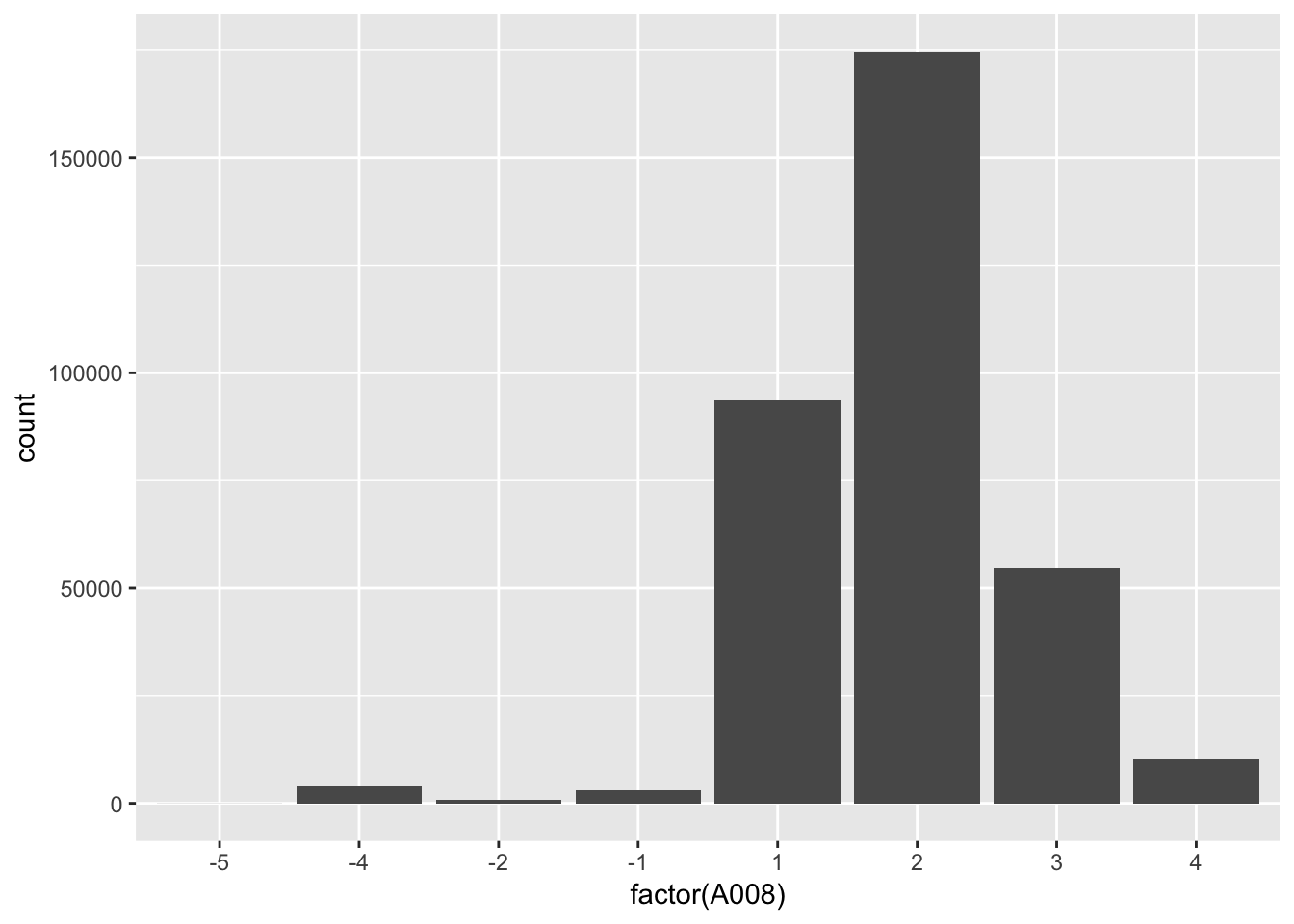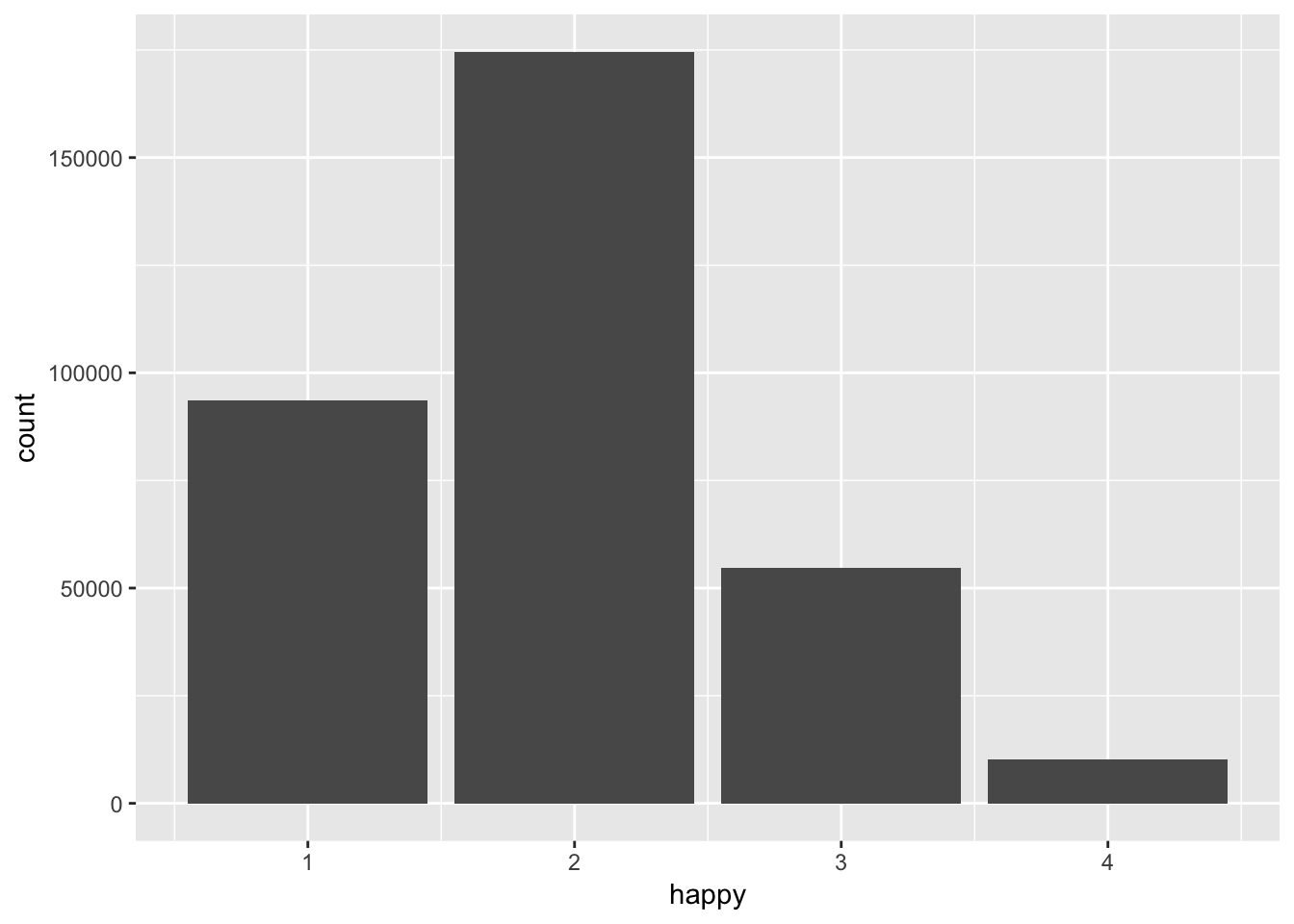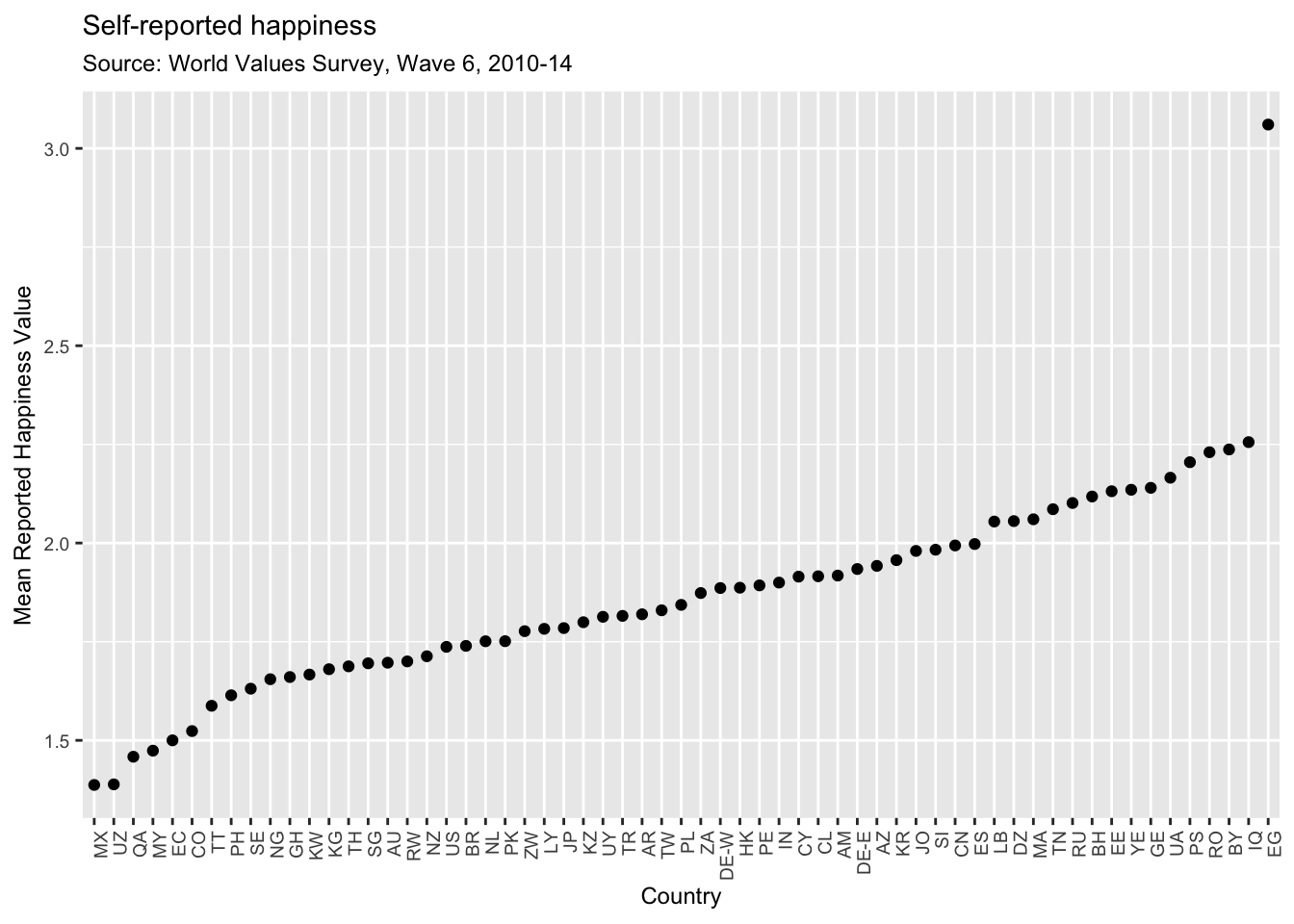``````library(tidyverse)
library(mosaic)``````

## The WVS Data

First, go to the World Value Survey site to see what we’ll be working with. Don’t download anything: http://www.worldvaluessurvey.org/WVSContents.jsp.

The World Value survey data is a BIG file. We have it accessible to us in a shared folder we can get through the R Studio Server. Alternatively, if you want to use RStudio on your laptop, you can download it from Moodle here:https://moodle.smith.edu/mod/resource/view.php?id=233901. If you download it from Moodle, you will then need to unzip the file in the folder you plan to use.

Once you have done that, you will notice that it is a kind of file that we haven’t used before, an .rdata file. These are data files specific to R. We haven’t ever had to load an .rdata file before, so let’s do that using the function `load()`.

The file’s name is “WVS_Longitudinal_1981_2014_R_v2015_04_18.Rdata” make sure you use the right file path to the data.

``load("/Users/openshare/WVS_Longitudinal_1981_2014_R_v2015_04_18.rdata")``

Running this command will take some time because the file is BIG! (really, it may take up to 5 minutes or so).

Now, to make it easier to work with, rename the data object:

``WVS <- WVS_Longitudinal_1981_2014_R_v2015_04_18``

What can we do to look at this data?

• Don’t just doubleclick on it to view it.
• Don’t type `View()`

We can consider some other ideas - `head()` the data

``head(WVS)``

What happens?

• What about finding out the structure of it with `str()`?
``str(WVS)``

What I would suggest you do is the following:

## What to do after looking at the codebook & questionnaire

See if you can do the following as an exercise:

• What I would suggest you do is think about whether you could `select()` a variable (or two) in which you’re interested.
• Can you then think about how you need to `mutate()` that variable to do some data analysis with it? (what are its values, are all of them useful?)
• Can you do a `favstats()` to get a sense of what it looks like? (remember to load the DataComputing package to do this.) What do you get if you can do that command? What would you want to look at otherwise?
• Could you do a `ggplot()` to do some kind of scatter plot/bar chart of the variable in which you’re interested? What would be a useful way to visualize the data?

## Case Study in Cleaning Data: Happiness

For example, here’s one variable, A008, for the question: “Taking all things together, would you say you are (fill in the value)

• Very happy
• Rather happy
• Not very happy
• Not at all happy" I looked in the codebook, and it’s listed as variable `A008`. So I look for it and select it, then head the data.
``````WVSfeelhappy <-
WVS %>%
select(A008)
``````##   A008
## 1    2
## 2    2
## 3    2
## 4    2
## 5    1
## 6    1``````

I can then run `favstats()` on the variable

``favstats(~A008, data = WVSfeelhappy)``
``````##  min Q1 median Q3 max     mean       sd      n missing
##   -5  1      2  2   4 1.837475 1.037906 341271       0``````

That doesn’t tell me much, because I need to edit the variable because it has values I can’t interpret like -5. I need to filter the data too. You could theoretically do that with your variable.

Notice, though, that I’ve lost all the country data. So I really need to `select()` another variable too before I only select A008. I want to have the variables for the country (S009A) and for the Wave of the Survey (S002). Working with a smaller data table is always much faster, so it’s easier just to select those three variables for now.

``````WVSfeelhappy <-
WVS %>%
select(S009A, S002, A008)``````

Now `head()` the data table and `favstats()` the variable A008. Again. Another tool I’d recommend to diagnose issues with data is just a straight plot of the data. We can plot the frequencies of the different reported values by using `geom_bar()` in `ggplot()`:

``````WVSfeelhappy %>%
ggplot(aes(x = factor(A008))) + #I tell it to treat A008 as a factor here
geom_bar()``````Notice we have values of -5 through -1 that aren’t too useful to us. We want to recode these as missing values by telling R they are NA.

We can do that by using `mutate()` in combination with the function `ifelse()` which is a logical string that says to test if something is true, then take a value if this is true, and another value if it is false.

So `ifelse(test, yes, no)`.

``````WVSfeelhappy <-
WVSfeelhappy %>%
mutate(happy = ifelse(A008 %in% c(-5, -4, -2, -1), NA, A008))``````

Now we’re going to ggplot again, but we don’t want a ggplot of the missing (NA) values for now, so we filter them out.

``````WVSfeelhappy %>%
filter(happy != is.na(happy)) %>%
ggplot(aes(x = happy)) +
geom_bar()``````Now that we have good values of the variable we want, we can now start to have fun with the data.

## Graphing mean happiness by country

For example, we can find the mean reported happiness grouping by country and the wave of the survey. Notice, though, that if someone doesn’t report a value, for the moment we’re going to tell R to ignore that missing value when computing the mean value of happiness. To do this, we tell R to add the option `na.rm = TRUE` to the call to the function `mean()`. This removes the missing values from the computation of the mean.

``````WaveCountryAves <-
WVSfeelhappy %>%
group_by(S009A, S002) %>%
summarise(meanhappy = mean(happy, na.rm = TRUE))
``````## # A tibble: 6 x 3
## # Groups:   S009A 
##   S009A  S002 meanhappy
##   <chr> <int>     <dbl>
## 2 AL        3      2.74
## 3 AL        4      2.41
## 4 AM        3      2.45
## 5 AM        6      1.92
## 6 AR        1      2.06``````

Now, let’s select the most recent wave of the survey, wave 6:

``````W6Happy <-
WaveCountryAves %>%
filter(S002 == 6)``````

And now we can plot the mean reported happiness for each country within the specific wave. Also, to make the figure inteligible I’ve reordered the x-axis by the mean value of mean reported happiness. If you’re interested, you can remove that part of the code to see what would happen instead. I’ve also added some additional details. I’ve asked `ggplot()` to switch the x-axis so that each label is at 90 degree to the axis and the size of the font is 9 points. I’ve also added a title and subtitle.

``````W6Happy %>%
ggplot(aes(x = reorder(S009A, meanhappy), y = meanhappy)) +
geom_point() +
theme(axis.text.x = element_text(angle = 90, hjust = 1),
text = element_text(size = 9)) +
xlab("Country") +
ylab("Mean Reported Happiness Value") +
labs(title = "Self-reported happiness",
subtitle = "Source: World Values Survey, Wave 6, 2010-14")``````Are the results of this for all countries credible? The values for Egypt (EG) are messing with our interpretation, so I’m going to filter Egypt, so I’m going to filter that country out for argument’s sake and re-do the figure. You can check the other country codes here.

``````W6Happy %>%
filter(S009A != "EG") %>%
ggplot(aes(x = reorder(S009A, meanhappy), y = meanhappy)) +
geom_point() +
theme(axis.text.x = element_text(angle = 90, hjust = 1),
text = element_text(size = 9)) +
xlab("Country") +
ylab("Mean Reported Happiness Value") +
labs(title = "Self-reported happiness",
subtitle = "Source: World Values Survey, Wave 6, 2010-14")``````